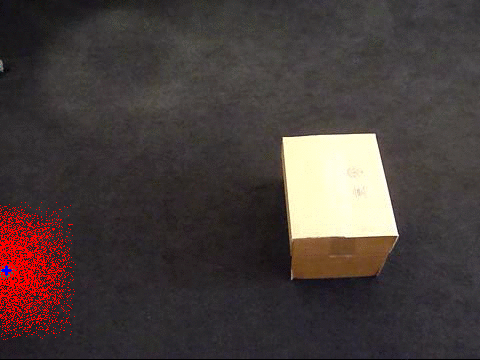## Thursday, 14 April 2016

### Particle Filtering - Survival of the fittest

I recently studied dynamic system models such as Kalman and Particle Filters.
For Kalman Filter I followed a Matlab demo that can be found here.

In this demo, the simple problem of tracking a ball is addressed using a Kalman Filter. The input sequence is of a ball, which is travelling at varying velocity and which is occluded in some frames by a box. I think this is a great example to demonstrate the power of dynamic system  models, especially the occluded frames can be used to test how good a dynamic model is. Here is the actual sequence:

As you can see the ball goes underneath the box and comes out of the other end. If our dynamic model is accurate it will be able to predict the state of the ball even when it is not visible, and should match the position when the ball comes out.

A Kalman Filter based modelling of the system can be seen at the Matlab demo page. Here I will present the results for a Particle Filter that I wrote. The purpose of this blog post is to give a high-level introduction to Particle Filter. A number of different implementation and detailed theory can be found very easily elsewhere.

A particle can be thought of a very small object having some randomness but at the same time having a particular state. In the above example, a particle that is defined by location, velocity and acceleration with its own randomness can be used. The main idea of Particle Filter is to only let the fittest particle survive - in this case, the particles that match the internal state of the ball would be considered as the fittest.

So I used a simple segmentation of the ball as my input:

The location of the ball is measured using simple blob analysis in Matlab. Once I have the measurements I can plug in my Particle Filter and see how the particles evolve:Particle Filter - Survival of the fittest

You can see each particle as red pixel, whereas the average state of all the particles is represented by blue +. I am not representing the weights of each particle, however these vary depending on how a particle fits into the system.

In the past, I have been fascinated by how randomness can be easily used to model complex systems in Random Forests, but this totally blows my mind - fascinating how each particle competes for being the model of the system and eventually only the fittest survive!!

References:
Object tracking using Kalman Filter: http://uk.mathworks.com/help/vision/examples/using-kalman-filter-for-object-tracking.html?requestedDomain=www.mathworks.com

Simple and awesome explanation of Particle Filter and the amazing importance resampling algorithm by Sebastian Thrun at: https://www.udacity.com/course/artificial-intelligence-for-robotics--cs373

Special thanks to my teacher Dr. Greg Slabaugh who explained Kalman Filter in a very awesome Computer Vision lecture.

Student Dave on Particle Filter: https://www.youtube.com/watch?v=O-lAJVra1PU